# Numeral Systems in Go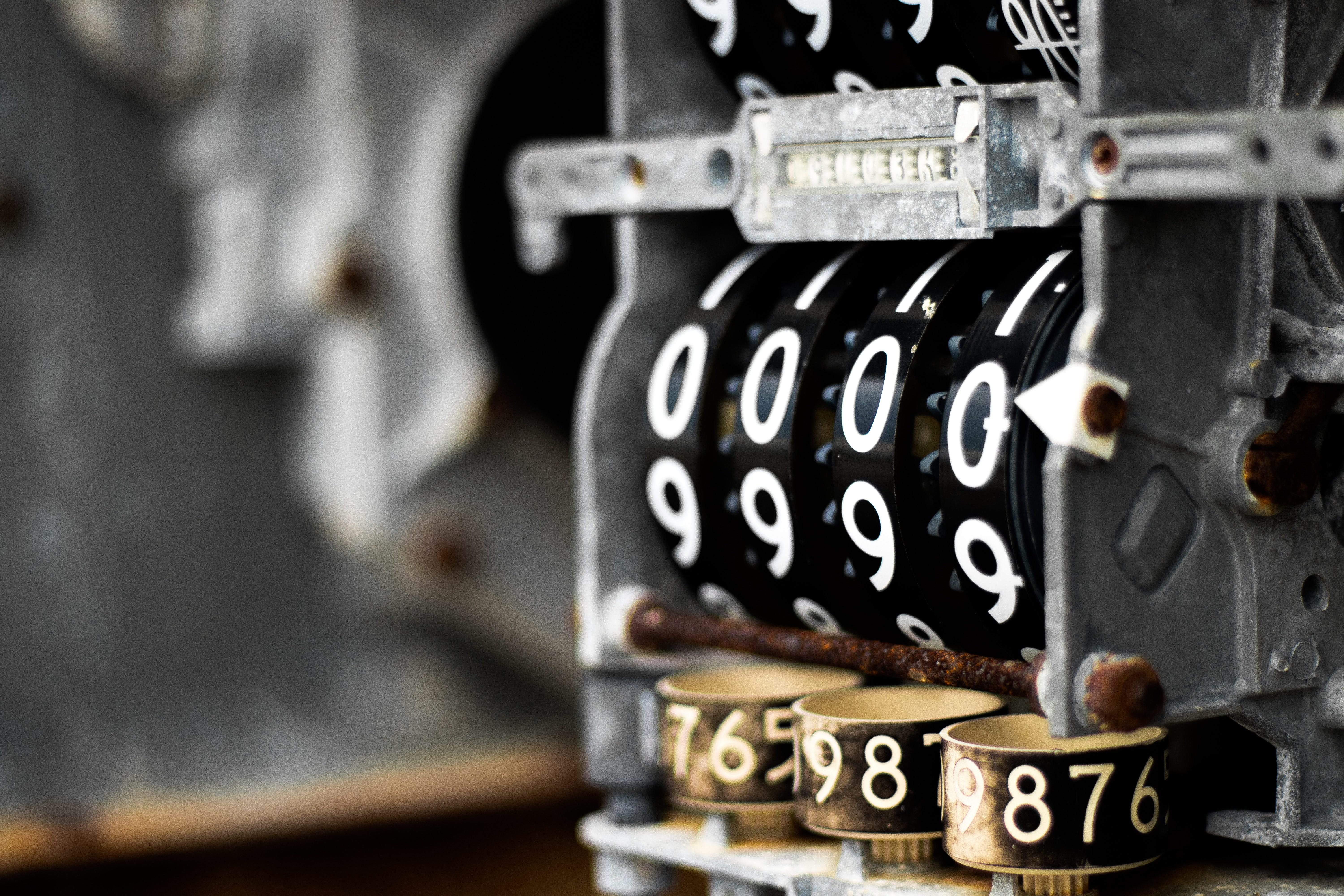photo by https://www.pexels.com/@logankir

A numeral is a symbol or group of symbols that represents a number. Numerals are not the same as numbers just as words are not the same with the things they refer to. The symbols “11(decimal)”, “1011(binary)” and “B(hexadecimal)” are different numerals, all representing the same number. The number is the idea, the numeral is anything that represents that idea.

A positional numeral system denotes usually the extension to any base of the Hindu–Arabic numeral system (or decimal system). In a more general sense, a positional system is a numeral system in which the contribution of a digit to the value of a number is the value of the digit multiplied by a factor determined by the position of the digit.

In modern positional systems, such as the decimal system, the position of the digit means that its value must be multiplied by some value: in 555, the three identical symbols represent five hundreds, five tens, and five units, respectively, due to their different positions in the digit string.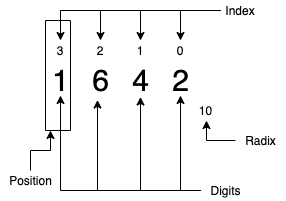Positional numeral systems glossary

According to its position of occurrence in the number, each digit is weighted. Towards the left, the weights increase by a constant factor equivalent to the base or radix. With the help of the radix point (‘.’), the positions corresponding to integral weights (1) are differentiated from the positions corresponding to fractional weights (<1).

Any integer value that is greater than or equal to two can be used as the base or radix.

The digit position ’n’ has weight r ^n. The largest value of digit position is always 1 less than the base value. The value of a number is a weighted sum of its digits.

For example:Decimal (0–9 digits with radix 10) 2056 value breakdownHexadecimal(0–9 & A-F digits with radix 16) 171B value breakdownThe general form of value breakdown, where b is the base or radix of the numeral system

# The Idea

Quick Link to package

I wanted to be able to implement any numeral system and play around with the representation of numbers without having to worry about the semantics of each system. At the end of the day, as we mentioned before multiple different numerals can represent the same number. So even operations between numerals from different systems shouldn’t be an issue when they can be translated to represent the same numbers.

For some reason, an image from the movie Imitation Game flashed in my head. The beloved moment in human history where Alan Turing’s machine, the bombe, came to life and the mechanical rotors started spinning.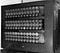A wartime picture of a Bletchley Park Bombe

A digit in any positional numeral system can be represented by the possible states it can take. Each state has a next state and a previous state. The next state of the last state is the first state while producing a carry-over.

A data structure like a circular list can effectively represent what we mentioned in the above paragraph.

In a doubly-linked circular list, the next pointer of the last node points to the first node, and the previous pointer of the first node points to the last node making the circular in both directions.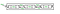doubly-linked circular list

Here comes Go’s ring. With the ring, we can easily represent any single digit of any positional numeral system by filling it with the possible ordered states and an initial value.

`// newDigit creates and initializes a new digit (ring).func newDigit(values []rune, state rune) (*ring.Ring, error) {   // initialize a new empty ring   r := ring.New(len(values))   // fill the ring with values   for _, e := range values {      r.Value = e      r = r.Next()   }   if indexOf(state, values) == -1 {      return nil, fmt.Errorf("invalid digit. value: %v does not exist in possible values: %v", state, values)   }   // roll the ring in desired "state" position.   for range values {      if r.Value == state {         break      }      r = r.Next()   }   return r, nil}`

What happens when a numeral contains more than one digit?
That can easily be solved by having a doubly-linked list whose elements would be consisted of doubly-linked circular lists.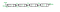doubly-linked list

This way we can easily create any positional representation of any number.

`// NewNumeral initializes a numeral by providing the initial number in strings// along with the possible values that each digit can have.func NewNumeral(values []rune, initial string) (*Numeral, error) {   // initialise a new number.   number := Numeral{      digits:      list.New(),      digitValues: values,   }   // add digits to the number along with their state.   for i := 0; i < len(initial); i++ {      digit, err := newDigit(values, rune(initial[i]))      if err != nil {         return nil, err      }      number.digits.PushBack(digit)   }   return &number, nil}`

Every time a digit resets back to the “first” state it would trigger a Next() to the next most valuable digit. Pretty simple right? In the case that our digits are all full and we want to increment, we can just create a new ring, initialize it and link it to the left side of our list.

Think of an example, a hypothetical numeral system with symbols [0–9,a-z] which has a radix of 36, and also imagine that we want to have a 4 “digit numeral” like “2z3r”.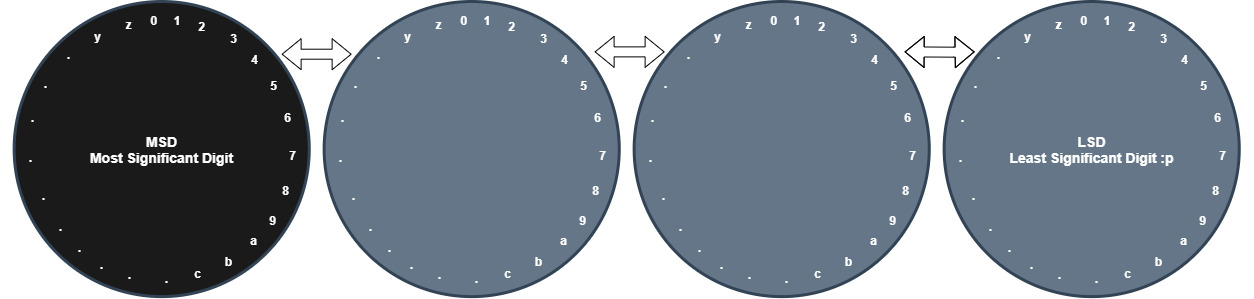visual representation of doubly-linked list of doubly-linked circular list
`// Numeral represents a numeral that is consisted by its digits// and digit values.type Numeral struct {   digits      *list.List   digitValues []rune}`

By defining a number like this all you need to have is a slice of the symbols you want to represent your number in the order they will have to exist.

`var values= []rune{'0', '1', '2', '3', '4', '5', '6', '7', '8', '9',   'a', 'b', 'c', 'd', 'e', 'f', 'g', 'h', 'i', 'j', 'k', 'l', 'm', 'n', 'o', 'p', 'q', 'r', 's', 't', 'u', 'v', 'w', 'x', 'y', 'z'}`

Then you need a “constructor” to generate numerals at will.

`// NewNumeral initializes a numeral by providing the initial number in strings// along with the possible values that each digit can have.func NewNumeral(values []rune, initial string) (*Numeral, error) {   // initialise a new number.   number := Numeral{      digits:      list.New(),      digitValues: values,   }   // add digits to the number along with their state.   for i := 0; i < len(initial); i++ {      digit, err := newDigit(values, rune(initial[i]))      if err != nil {         return nil, err      }      number.digits.PushBack(digit)   }   return &number, nil}`

Initializing a numeral after we have reached this point is pretty straightforward.

`number, _ := numeral.NewNumeral(values, "2z3r")`

# Performing Simple Operations

This approach makes +1 and -1 operations very fast since lists (either circular or not) always know their next and previous node.

For example:

• Incrementing a Hexadecimal numeral “12d4” by one needs to execute 1 next() to the rightmost ring, that's all. The result is “12d5”.
• Incrementing a Hexadecimal numeral “dfff” by one needs to execute 4 next() calls on the 3 rightmost rings and one last on the 4th most significant ring. The result is “e000”.
• Decrementing`1`will operate in a similar way.
`// Increment performs a +1 to the Numeral.func (n *Numeral) Increment() error {   // take the first digit from the right and keep going if there are any arithmetic holdings.   for e := n.digits.Back(); e != nil; e = e.Prev() {      // get current ring.      r := e.Value.(*ring.Ring)      // rotate and update.      r = r.Next()      e.Value = r      // if the digit is not being reset (no arithmetic holdings) then there is no need to      // proceed in adding on the others.      if r.Value != n.digitValues {         break      }      // If needed add an extra new digit on the left side.      if e.Prev() == nil {         d, _ := newDigit(n.digitValues, n.digitValues)         n.digits.PushFront(d)      }   }   return nil}`

Adding and Subtracting can also happen efficiently even between numerals that are based on different systems by using the most known numbers of the decimal system that our computers easily understand.

• Adding 2 numerals will just require converting both of those to decimal integers, add them and generate a new numeral from the result. The result numeral can even be on a different numeral system easily by generating it from an integer.

Converting any numeral to integer is easy by performing a value breakdownthe general form of value breakdown, where b is the base or radix of the numeral system
`// Decimal converts a numeral to a decimal integer.func (n *Numeral) Decimal() int {   dec := 0   di := 0   for d := n.digits.Back(); d != nil; d = d.Prev() {      // get current ring.      r := d.Value.(*ring.Ring)      // get the index of the ring.      i := indexOf(r.Value.(rune), n.digitValues)      // Add digit's decimal counterpart to the decimal sum.      dec = dec + i*powInt(len(n.digitValues), di)      di++   }   return dec}`

# Let’s Talk Numbers

Running some benchmarks on the Increment() method for 3 different numeral systems for different initial values (small and big) results in some very nice results. The average execution time of the Increment function for binary numerals after 1 billion increments is around 7.6ns / op.

As one may expect, selecting a numeral system with a higher numeral range results in carryovers appearing less frequently, therefore the transitions that need to happen are fewer (increments/decrements)which results in better performance.

`goos: linuxgoarch: amd64pkg: github.com/slysterous/numeralcpu: Intel(R) Core(TM) i7–7700 CPU @ 3.60GHzBenchmarkNumeralIncrementDecimalSmall-8 1000000000 4.604 ns/opBenchmarkNumeralIncrementDecimalLarge-8 1000000000 4.574 ns/opBenchmarkNumeralIncrementBinarySmall-8 1000000000 7.644 ns/opBenchmarkNumeralIncrementBinaryLarge-8 1000000000 7.655 ns/opBenchmarkNumeralIncrementHexSmall-8 1000000000 4.427 ns/opBenchmarkNumeralIncrementHexLarge-8 1000000000 4.652 ns/op`

How about converting numerals into decimal integers? From the benchmarks that I performed on Hex and Binary numerals, the process takes less than 1 μsecond. The opposite process, converting from decimal ints to any numeral is similar.

Since converting to decimals requires traversing through every digit to sum its weight, it’s easy to reach the conclusion that the more digits there are, the slower the process will be. This can also be reflected in the below benchmark.

`go test -bench=NumeralDecimal -benchtime=60sgoos: linuxgoarch: amd64pkg: github.com/slysterous/numeralcpu: Intel(R) Core(TM) i7–7700 CPU @ 3.60GHzBenchmarkNumeralDecimalFromHex0–8 1000000000 8.182 ns/opBenchmarkNumeralDecimalFromHexfffff-8 252367634 284.8 ns/opBenchmarkNumeralDecimalFromHexffffffffff-8 250184980 286.0 ns/opBenchmarkNumeralDecimalFromHexffffffffffffffffffff-8 100000000 616.6 ns/opBenchmarkNumeralDecimalFromBinary0–8 1000000000 7.818 ns/opBenchmarkNumeralDecimalFromBinary10000–8 837013039 84.98 ns/opBenchmarkNumeralDecimalFromBinary1000000000–8 337445121 214.8 ns/opBenchmarkNumeralDecimalFromBinary1000000000000000000–8 158028769 455.9 ns/op`

Alright, one last check. How does adding numerals of different systems perform? Remember, I’m using decimal integers as the intermediate common base to perform operations. Turns out adding is also relatively fast since it takes roughly around 8 μseconds to perform an average addition

Additions are a bit trickier in terms of performance. At least 2 conventions have to happen, both numbers are translated to decimal and in the end, the result is converted back to the final desired numeral system. This relies heavily on the performance of the conversion of a numeral to decimal int.

`go test -bench=NumeralAdd -benchtime=60sgoos: linuxgoarch: amd64pkg: github.com/slysterous/numeralcpu: Intel(R) Core(TM) i7–7700 CPU @ 3.60GHzBenchmarkNumeralAddBinaryOnHex-8 9691966 8651 ns/opBenchmarkNumeralAddHexOnHex-8 9144648 8290 ns/opBenchmarkNumeralAddDecOnDec-8 11384712 7014 ns/opBenchmarkNumeralAddSumBinaryOnHex-8 11281405 6237 ns/opBenchmarkNumeralAddSumHexOnHex-8 12745245 5873 ns/opBenchmarkNumeralAddSumDecOnDec-8 12672326 5849 ns/op`

In conclusion, numeral is now a Go package. It gives you the ability to create custom (positional) numeral systems and perform operations on them. Feel free to contribute and don’t hesitate to reach out for any questions.